# Choose State-Space Model Specification Using Backtesting

This example shows how to choose the state-space model specification with the best predictive performance using a rolling window. A rolling window analysis for an explicitly defined state-space model is straightforward, so this example focuses on implicitly defined state-space models.

Suppose that the linear relationship between the change in the observed unemployment rate and the nominal gross national product (nGNP) growth rate is of interest. Suppose further that you want to choose between an AR(1) or an AR(2) model for the first difference of the unemployment rate (i.e., the state). That is,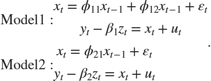•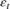and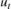are Gaussian process with mean 0 and variance 1.

•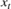is the true unemployment rate at time.

•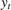is the observed unemployment.

•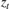is the nGNP rate.

Declare the functions rwParamMap.m and rwAR2ParamMap.m, which specify how the parameters in params map to the state-space model matrices, the initial state values, and the type of state. Save them in your working folder.

function [A,B,C,D,Mean0,Cov0,StateType,deflateY] = rwParamMap(params,y,Z) %rwParamMap Parameter-to-matrix mapping function for rolling window example %using ssm % The state space model specified by rwParamMap contains a stationary % AR(1) state, the observation model includes a regression component, and % the variances of the innovation and disturbances are 1. The response y % is deflated by the regression component specified by the predictor % variables x. A = params(1); B = 1; C = 1; D = 1; Mean0 = []; Cov0 = []; StateType = 0; deflateY = y - params(2)*Z; end 
function [A,B,C,D,Mean0,Cov0,StateType,deflateY] = rwAR2ParamMap(params,y,Z) %rwParamMap Parameter-to-matrix mapping function for rolling window example %using ssm and specifying an ARMA state model % The state space model specified by rwParamMap contains a stationary % AR(2) state, the observation model includes a regression component, and % the variances of the innovation and disturbances are 1. The response y % is deflated by the regression component specified by the predictor % variables x. A = [params(1) params(2); 1 0]; B = [1; 0]; C = [1 0]; D = 1; Mean0 = []; Cov0 = []; StateType = [0 0]; deflateY = y - params(3)*Z; end 

Load the Nelson-Plosser data set, which contains the unemployment rate and nGNP series, among other things.

load Data_NelsonPlosser 

Preprocess the data by taking the natural logarithm of the nGNP series, and the first difference of each. Also, remove the starting NaN values from each series.

isNaN = any(ismissing(DataTable),2); % Flag periods containing NaNs gnpn = DataTable.GNPN(~isNaN); ur = DataTable.UR(~isNaN); % Sample size Z = diff(log(gnpn)); y = diff(ur); T = size(y,1); 

If you define a state-space model implicitly and the response and predictor data (i.e., y and Z) exist in the MATLAB® Workspace, then the software creates a link from the parameter-to-matrix mapping function those series. If the data do not exist in the MATLAB® Workspace, then the software creates the model, but you must provide the data using the appropriate name-value pair arguments when you, e.g., estimate the model.

Therefore, to conduct a rolling window analysis when the state-space model is implicitly defined and there is a regression component, you must specify the state-space model indicating the indices of the data to be analyzed for each window. Conduct a rolling window analysis of the simulated data. Let the rolling window length (m) be 40 periods and the forecast horizon (h) be 10 periods. For this example, assume that the time-series are stable (i.e., all parameters are time-invariant).

m = 40; N = T - m + 1; % Number of rolling windows h = 10; fError1 = nan(N,h); % Preallocation fError2 = nan(N,h); for j = 1:N; idxRW = j:(m + j - h - 1); idxFH = (m + j - h):(m + j - 1); Mdl1 = ssm(@(c)rwParamMap(c,y(idxRW),Z(idxRW))); Mdl2 = ssm(@(c)rwAR2ParamMap(c,y(idxRW),Z(idxRW))); [EstMdl1,estParams1] = estimate(Mdl1,y(idxRW),[0.5 -20]',... 'Display','off'); [EstMdl2,estParams2] = estimate(Mdl2,y(idxRW),[0.5 0.1 -20]',... 'Display','off'); fY1 = forecast(EstMdl1,h,y(idxRW),'Predictors0',Z(idxRW),... 'PredictorsF',Z(idxFH),'Beta',estParams1(end)); fY2 = forecast(EstMdl2,h,y(idxRW),'Predictors0',Z(idxRW),... 'PredictorsF',Z(idxFH),'Beta',estParams2(end)); fError1(j,:) = y(idxFH) - fY1; fError2(j,:) = y(idxFH) - fY2; end 

Compute the RMSE for each step-ahead forecast, and compare them for each model.

fRMSE1 = sqrt(mean(fError1.^2)); fRMSE2 = sqrt(mean(fError2.^2)); fRMSE1 < fRMSE2 
ans = 1x10 logical array 1 1 1 0 0 0 0 0 0 0 

Overall, the predictive performance of the AR(2) model is better than the AR(1) model.

Alternatively, you can compare the predictive performance of the models using the Diebold-Mariano test. For more details, see .